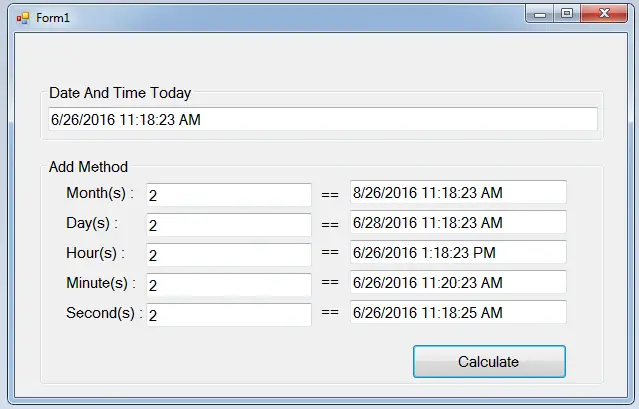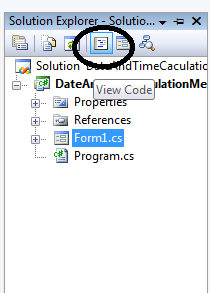# Date and Time Calculation Methods In C#

## Date and Time Calculation Methods In C#

Date and Time Calculation Methods in C#

Now, you are going to learn how to calculate the date and time of the present date using C#.net. This tutorial will help you calculate the months, days, hour, minutes and seconds of a present date in an instant. As a result, the numbers of the precise time will be displayed accurately in each field.## So, let’s begin:• Go to the solution explorer, click the “view code” to fire the code editor.• Create a method to calculate today’s date to future date.
```private void Calculate(){
//'INITIALIZE THE INSTANCE METHODS OF THE DATETTIME TYPE.

//declare the date today
DateTime dateNow = DateTime.Now;
//declare the string variable
string ansMon,ansDay,ansHr,ansMin,ansSec;
//set the date today into the textbox
txtDateAndTimeToday .Text = dateNow.ToString();

//passing the added months,days,hours,minutes and seconds to the string variable.

//set up the added months,days,hours,minutes and seconds to display in the textboxes
txtAnsMonths.Text = ansMon;
txtAnsDays.Text = ansDay;
txtAnsHours.Text = ansHr;
txtAnsMinutes.Text = ansMin;
TxtAnsSeconds.Text = ansSec;

}```
• Go back to the design view, double-click the form and call a method to calculate today’s date to future date in the first load of the form.
``` private void Form1_Load(object sender, EventArgs e)
{
//'SET THE METHOD TO CALCULATE IN THE FIRST LOAD
Calculate();
}```
• Go back to the design view again, double-click the “calculate” button to fire the <code> click</code>  event handler of a button and do the following code in the method.
```private void button1_Click(object sender, EventArgs e)
{
//'SET THE METHOD TO CALCULATE WHEN BUTTON IS CLICKED
Calculate();
}```

### Output:For all students who need programmer for your thesis system or anyone who needs a source code in any programming languages. You can contact me @ :

Email – [email protected]
Mobile No. – 09305235027 – tnt

Date and Time Calculation Methods